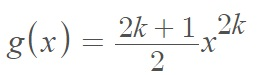# Standard Power Distribution & U Power Distribution

Share on

## Standard Power Distribution

The standard power distribution has the probability density function f(x) = ΒxΒ-1; 0 < x < 1.
The distribution has one shape parameter, Β.

The distribution isn’t found very often in the literature, possibly because it isn’t a realistic model for real-world applications. One application is in random number generation, where random samples are drawn from the function .

## U-Power Distribution

The standard U-power distribution is a U-shaped, continuous probability distribution with one shape parameter k &isin ℕ. The distribution, which is defined on the interval [-1, 1] is based on a family of power functions which take on the distinctive u-shape .

The U-power distribution has the probability density function:.
In general, the PDF is monotone increasing with a global maximum occurring at the upper boundary of the domain (x = 1 for the standard distribution). The shape parameter controls the overall shape, including height, spread, and location of its maximum point.
When k = 1 (graphed in red in the above image), the distribution is called the U-quadratic distribution. When k = 0, the distribution reduces to the uniform distribution, which is not u-shaped.

The distribution function has a few notable properties:

• Symmetry about x = 0,
• Concave up (i.e,. a right-side-up-U),
• Modes at x = ±1,
• Decreasing for x < 0 and increasing for x>0.
• Minimum at x = 0.

Related article: Exponential Power Distribution.

## References

Graph created with Desmos.
 numpy.random.power.
 Siegrist, K. 5.26: The U Power distribution. Retrieved December 31, 2021 from: https://stats.libretexts.org/Bookshelves/Probability_Theory/Probability_Mathematical_Statistics_and_Stochastic_Processes_(Siegrist)/05%3A_Special_Distributions/5.26%3A_The_U-Power_Distribution

CITE THIS AS:
Stephanie Glen. "Standard Power Distribution & U Power Distribution" From StatisticsHowTo.com: Elementary Statistics for the rest of us! https://www.statisticshowto.com/standard-power-distribution-u-power-distribution/
---------------------------------------------------------------------------Need help with a homework or test question? With Chegg Study, you can get step-by-step solutions to your questions from an expert in the field. Your first 30 minutes with a Chegg tutor is free!